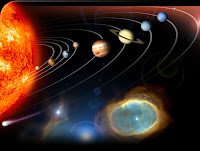How far other planets from the earth: How to calculate distance of other planetsIf we think about how far other planets from earth than some how you will get difficult to find out this answers. Most of the people do not know that how far other solar system planet from our earth due to the planets are moving in theirs own orbit all time which make variable distance of every planet from the Planet earth. Here I am giving short data which help you to know how the planets are farther from our Earth and you also will be able to know which planet is closest planet to the Earth. It is difficult to calculate the distance of other planet in our normal unit so the scientist have introduced a unit to measure the heavenly bodies distances. Generally all the heavenly bodies use to be calculate in Astronomical Unit (AU). The Astronomical Unit is the distance from the Earth to the Sun and which is nearly about 150 million kilometers or 93 million miles. Followings are the AU distance of every planets

Planet Distance from Sun in AUMercury 0.39
Venus 0.72
Earth 1.0
Mars 1.5
Jupiter 5.2
Saturn 9.5
Uranus 19.2
Neptune 30.1
Pluto 39.5

For finding the distance between orbits of the two Planets in Astronomical Unit, subtract two planet- sun distance. Suppose the Earth orbits at the one AU from Sun and the Venus orbits at 0.72 AU from the Sun. Difference between these 2 distances is 1-0.72 which is equal to 0.28 AU. And for finding out the number in Kilometer simply multiply by conversion above 0.28 X 150=42 km. Let's have another example Jupiter is 5.2 AU from the sun and the earth is 1 AU from the sun. So now the calculation of the distance between the Jupiter orbit and the earth orbit will be 5.2-1= 4.2 AU and in kilometer it will be 630 million km. So following above are the simple process to calculate the distance.We also have calculate the Mars distance in 2003 August, as we know that fairly Planet Mars have elliptical shaped orbit that meant its "closest approach" distance change. At the time of August 2003 Mars was closer to our Earth which have been only in about 60,000 years. The numbers above given are used in introductory astronomy classes.